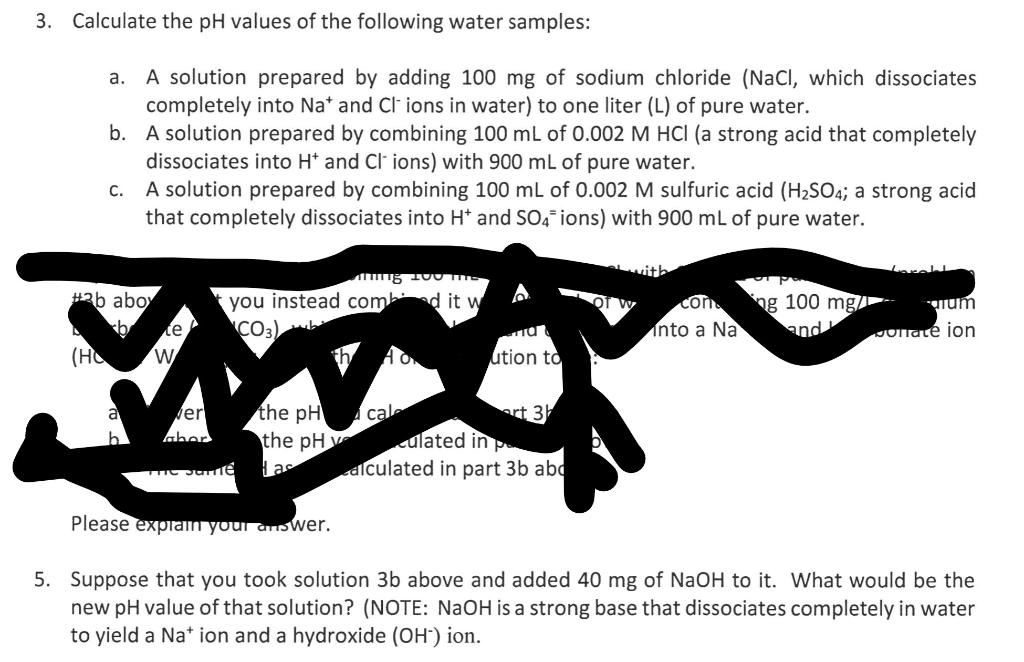# (Solved): Q 3b and 5 please 3. Calculate the $$\mathrm{pH}$$ values of the following water samples: a. A so ...Q 3b and 5 please

3. Calculate the $$\mathrm{pH}$$ values of the following water samples: a. A solution prepared by adding $$100 \mathrm{mg}$$ of sodium chloride $$(\mathrm{NaCl}$$, which dissociates completely into $$\mathrm{Na}^{+}$$and $$\mathrm{Cl}^{-}$$ions in water) to one liter ( $$\mathrm{L}$$ ) of pure water. b. A solution prepared by combining $$100 \mathrm{~mL}$$ of $$0.002 \mathrm{M} \mathrm{HCl}$$ (a strong acid that completely dissociates into $$\mathrm{H}^{+}$$and $$\mathrm{Cl}^{-}$$ions) with $$900 \mathrm{~mL}$$ of pure water. c. A solution prepared by combining $$100 \mathrm{~mL}$$ of $$0.002 \mathrm{M}$$ sulfuric acid $$\left(\mathrm{H}_{2} \mathrm{SO}_{4}\right.$$; a strong acid that completely dissociates into $$\mathrm{H}^{+}$$and $$\mathrm{SO}_{4}=$$ ions) with $$900 \mathrm{~mL}$$ of pure water. Suppose that you took solution $$3 \mathrm{~b}$$ above and added $$40 \mathrm{mg}$$ of $$\mathrm{NaOH}$$ to it. What would be the new $$\mathrm{pH}$$ value of that solution? (NOTE: $$\mathrm{NaOH}$$ is a strong base that dissociates completely in water to yield a $$\mathrm{Na}^{+}$$ion and a hydroxide $$\left(\mathrm{OH}^{-}\right)$$ion.

We have an Answer from Expert